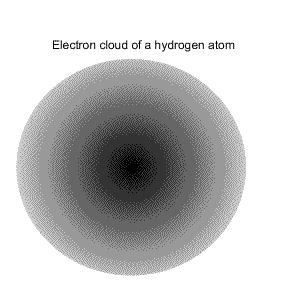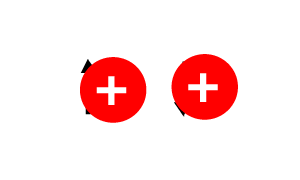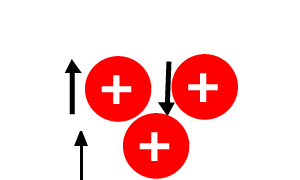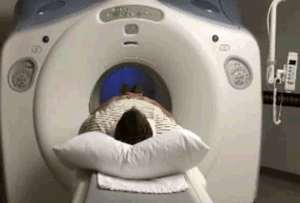NMR
Nuclear magnetic resonance spectroscopy (NMR) has become the main technique for determining the structure of organic molecules. NMR uses larger quantities, roughly 1 milligram, of a substance than mass spectroscopy and is non-destructive.

A spinning charge generates a magnetic field. Protons, neutrons and electrons spin on their axis in either an up or down configuration. This spinning of charge creates a very small magnetic field.

As shown on the right, the proton in a hydrogen atom spins on its axis.Where the nucleus contains more than one nucleon their spins are paired so that they cancel out.However, in a nucleus with an odd number of nucleons the nucleus has a permanent overall spin. This is why atoms, such as the 1H and the 13C are used in nmr.Subatomic particles such as electrons, protons and neutrons are thought of as spinning on their axes. In many atoms, such as 12C, these spins are paired against each other so that they cancel each other out and so the nucleus of the atom has no overall spin. In some atoms, with an odd number of nucleons, such as 1H and 13C the nucleus does have an overall spin. How do we determine if a nucleus will have an overall spin? Follow the rules below.
1. If the number of neutrons and the number of protons are both even, then the nucleus has no overall spin.
2. If the atomic mass is an odd number, such as 15N, then the nucleus has a half-integer spin (i.e. 1/2, 3/2, 5/2)
3. If the atomic mass is an even number and the number of protons is an odd number, as in 10B, then the nucleus has an integer spin (i.e. 1, 2, 3).

View the video on the right.NMR is used as a diagnostic tool. It can produce a three dimensional view of the internal body. This can be extremely useful for doctors diagnosing cancer or faulty blood vessels.

View a 3d image of the brain.

Continue